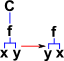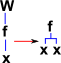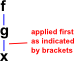# Maths - Combinatory Logic

This was introduced by Moses Schnfinkel and Haskell Curry with the aim of eliminating the need for variables in mathematical logic. It is equivalent to lambda calculus but it can be used for doing, without variables, anything that would require variables in other systems.

#### OperatorsWe can think of functions as taking some 'value' and modifying it in some way to give a potentially different value. Operators raise this up a level so functions become the elements and operators act on the functions.

There are many examples of operators in mathematics, for example in calculus, integration and differentiation take a function and return a different, but related, function.

As the name 'combinators' suggests here we are interested in the ways that existing functions can be combined to produce new functions. For example, a possible way to combine two functions is function composition, that is: if we have two functions f and g then we can combine them by applying f first and then applying g to the output of f (provided the types are compatible of course). Here are some possible ways to combine functions:

Operator What it does Expression Lambda
Equivalent
SKI
equivalent
(normal form)
I Identity (leave unchanged) I(f) = f

λx.x
Ix->x

I or SKK or SKS
B Function composition (B(f,g))(x) = f(g(x)) λxyz.x(yz) S(KS)K
B' Reverse function composition (B'(f,g))(x) = g(f(x)) λxyz.y(xz)
C Swap functions (C(f))(x,y) = f(x,y) λxyz.xzy S(K(SI))K
K Form constant function ((K f) g) = f λxy.x
Kxy->x
K
S Generalized composition (S f g x) = f(x,g(x)) λxyz.xz(yz)
Sxyz->xz(yz)
S
W Doubling or diagonalizing (W(f))(x) = f(x,x) λxy.xyy

Where:

• x,y,z: are elements
• f, g, h: are functions on those elements
• upper case letters are operators

### Simplifying Operator Expressions

Often what we want to do is separate out the elements x,y,z so we get something like this:

O (x,y) = P (x,y)

Where O and P are some combination of operators and functions.

So that we can effectively cancel out the elements:

O = P

So we have stepped up a level and are operating purely in terms of functions. When discussing this for functors on the category theory pages we described this as 'lifting'.

An example of this is the axioms of algebra such as the commutative law, usually this is written in terms of arbitrary variables like this:

 (x,y) x + y = y + x

Or if we use + as a prefix rather than an infix operator:

 (x,y) +(x,y) = +(y,x) +(x,y) = (C+)(x,y)

Where C means: swap functions as explained in the table above.

So we can cancel out the elements (x,y) and just represent the commutative axiom as:

+ = C(+)

So using operators we don't have to describe axioms in terms of arbitrary elements.

redex is short for reducible expression

### Combining Combinators

So far we have applied combinators to functions and elements. But how do the combinators interwork? I find it helps to think of combinators as modifiers of a tree structure. In this tree structure elements (x, y ...) are leaves of the tree, functions may either be leaves or nodes. So in this system the combinators look like this:

 tree diagram expressionIX=X KXY = X SXYZ =(X Z(Y Z))

So in this system, how do we represent multiple combinators such as: SKK?((S K K) x) = (S K K x) = (K x (K x)) = x

So here we have used the following conventions:

Diagram Notation Text Notation
function call:(f x)
the function is shown as the first symbol inside the brackets, in this case 'f'.
parameters for function :(f x y)
the parameters are the second and remaining symbols inside the brackets, in this case 'x' and 'y'.
function composition:(f (g x))
here f is applied to the result of the inner function g. So 'g x' is distinguished from being two parameters of 'f' by the inner brackets.
default precedence:if we have no brackets, for example:
A B C
then this implies brackets from the left:
(A B) C
That is A is applied to B and the result of this is applied to C. If we want A to be applied to the result of B applied to C then we must explicitly include the brackets like this:
A (B C)
Also, if we want B and C to be parameters for A then we must explicitly include the brackets around the whole function, like this:
(A B C)

#### Example - Equivalent SKI sequence for C

 C x y =S(K(SI))K x y =K(SI)x(Kx)y =SI(Kx)y =Iy(Kxy) =Iyx =yx

### The Curry-Howard Isomorphism

Combinatory Logic Intuitional Logic
logic types (function type signatures) theorems
programs programs (typed lambda term) proofs of those theorems

This gives an isomorphism where theorems in intuitionistic logic correspond to type signatures in combinatory logic and programs in intuitionistic logic correspond to the proofs of those theorems in combinatory logic.

### K and S Combinators

Here are some combinators, all others can be derived from K and S.

Sometimes known as SKI calculus.

Combinator Function Definition Type Signature (Axiom)
I = identity (I x) = x
for all terms x
do nothing (leave unchanged)
K ((K x) y) = x

manufactures constant functions (for any argument, return x):

K: A -> (B -> A)

S (S x y z) =
(x z (y z))

generalized version of application

S applies x to y after first substituting z into each of them. Or put another way, x is applied to y inside the environment z.

S: (A -> (B -> C)) -> ((A -> B) -> (A -> C))

MP

function application corresponds to the detachment (modus ponens) rule:

from A and A -> B infer B

I and MP are not strictly necessary because all types can be represented with come combination of S and K. for example I is equivalent to:

```((S K K) x)
= (S K K x)
= (K x (K x))
= x
```

These are only 'equivalent' not 'equal' or 'identical' because they produce the same result for the same arguments but they are not the same.

## De Bruijn index

SKI Operator λ-calculus De Bruijn index
K λx. λy. x λ λ 2
S λx. λy. λz. x z (y z) λ λ λ 3 1 (2 1)

## Modeling Computation

 metadata block see also: Formal Systems Stanford Encyclopedia of Philosophy wiki1 wiki2 Youtube video Advanced Topics in Programming Languages Series: Parametric Polymorphism and the Girard-Reynolds Isomorphism. This talk is based on a series of papers by Philip Wadler, a principal designer of the Haskell programming language. Featured are a number of double-barreled names in computer science: Hindley-Milner (Strong typing without having to type the types) Wadler-Blott (Making ad-hoc polymorphism less ad-hoc with parametricity) Curry-Howard (Isomorphism between types and theorems, terms and proofs) Girard-Reynolds (Isomorphism between types and terms in the presence of parametricity) or Djinn code see here. Parametricity Correspondence about this page

This site may have errors. Don't use for critical systems.